## 14.2.2 The Discrete-Time Model

This section introduces a simple and effective way to sample the space of action trajectories. Section 14.2.3 covers the more general case. Under differential constraints, sampling-based motion planning algorithms all work by sampling the space of action trajectories. This results in a reduced set of possible action trajectories. To ensure some form of completeness, a motion planning algorithm should carefully construct and refine the sample set. As in Chapter 5, the qualities of a sample set can be expressed in terms of dispersion and denseness. The main difference in the current setting is that the algorithms here work with a sample sequence over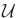, as opposed to overas in Chapter 5. This is required because solution paths can no longer be expressed directly on(or).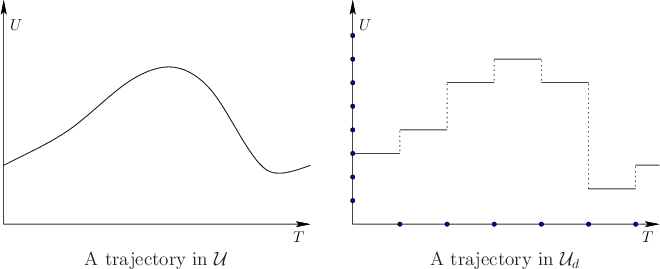The discrete-time model is depicted in Figure 14.5 and is characterized by three aspects:

1. Time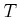is partitioned into intervals of length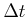. This enables stages to be assigned, in which stageindicates that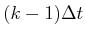units of time have elapsed.
2. A finite subset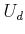of the action space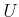is chosen. Ifis already finite, then this selection may be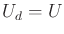.
3. The action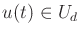must remain constant over each time interval.
The first two discretize time and the action spaces. The third condition is needed to relate the time discretization to the space of action trajectories. Let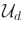denote the set of all action trajectories allowed under a given time discretization. Note thatcompletely specifies the discrete-time model.

For some problems,may already be finite. Imagine, for example, a model of firing one of several thrusters (turn them on or off) on a free-floating spacecraft. In this case no discretization ofis necessary. In the more general case,may be a continuous set. The sampling methods of Section 5.2 can be applied to determine a finite subset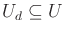.

Any action trajectory incan be conveniently expressed as an action sequence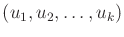, in which each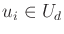gives the action to apply from time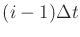to time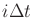. After stage, it is assumed that the termination action is applied.

Subsections
Steven M LaValle 2020-08-14